# Dual basis

(diff) ← Older revision | Latest revision (diff) | Newer revision → (diff)
Jump to: navigation, search

to a basisof a modulewith respect to a formA basis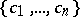ofsuch that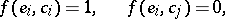whereis a free-module over a commutative ringwith a unit element, andis a non-degenerate (non-singular) bilinear form on.

Let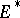be the dual module of, and let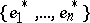be the basis ofdual to the initial basis of: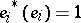,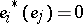,. To each bilinear formonthere correspond mappings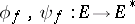, defined by the equationsIf the formis non-singular,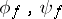are isomorphisms, and vice versa. Here the basisdual to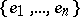is distinguished by the following property: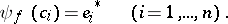#### Comments

A bilinear formonis non-degenerate (also called non-singular) if for all,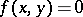for allimpliesand for all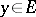,for allimplies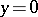. Occasionally the terminology conjugate module (conjugate space) is used instead of dual module (dual space).

How to Cite This Entry:
Dual basis. Encyclopedia of Mathematics. URL: http://encyclopediaofmath.org/index.php?title=Dual_basis&oldid=14785
This article was adapted from an original article by E.N. Kuz'min (originator), which appeared in Encyclopedia of Mathematics - ISBN 1402006098. See original article